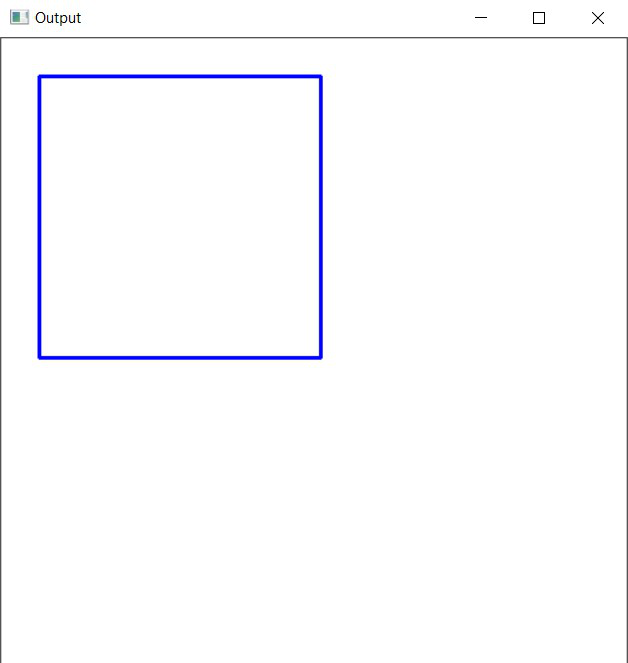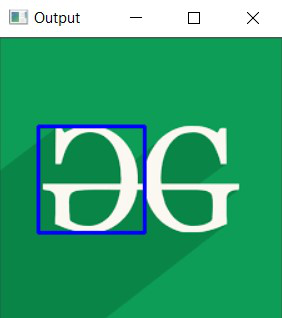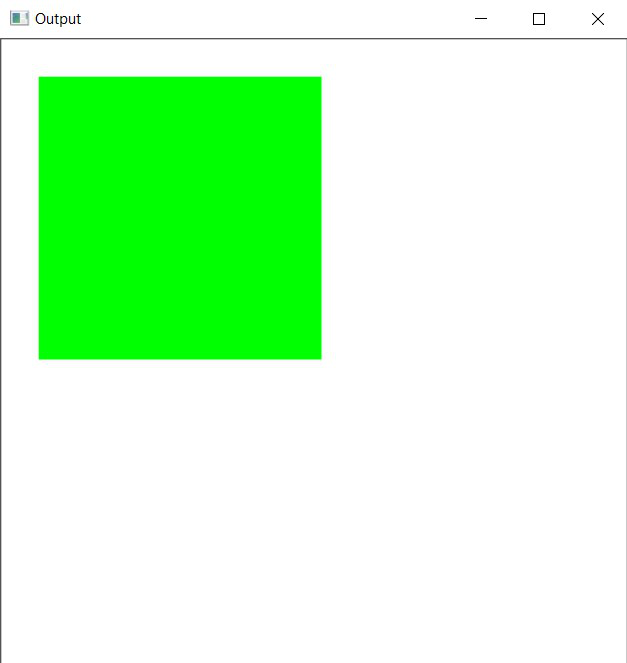GeeksforGeeks App
Open AppBrowser
Continue

# Draw an rectangle using OpenCV in C++

In this article, the task is to draw an rectangle using OpenCV in C++. The rectangle() function from OpenCV C++ library will be used.

Syntax:

rectangle( img, pt1, pt2, color, thickness, line Type, shift)

Parameters:

• image: It is the image on which the rectangle is to be drawn.
• start(pt1): It is the top left corner of the rectangle represented as the tuple of two coordinates i.e., (x-coordinate, y-coordinate).
• end(pt2): It is the bottom right corner of the rectangle represented as the tuple of two coordinates i.e., (x-coordinate, y-coordinate).
• color: It is the color of the borderline of the rectangle to be drawn. A tuple representing 3 colors (B, G, R) i.e., (Blue, Green, Red).
• thickness: It is the thickness of the rectangle borderline in px. The thickness of -1 px will fill the rectangle shape by the specified color.
• lineType: Type of the line. There are 3 types of line:
• LINE_4: Line was drawn using 4 connected Bresenham algorithm.
• LINE_8: Line was drawn using 8 connected Bresenham algorithm.
• LINE_AA: It draws Anti-aliased lines formed by using a Gaussian filter.
• shift: The number of fractional bits in the point coordinates.

Return Value: It returns an image.

Program 1:

Below is the C++ program demonstrating how to draw a rectangle over a self-formed background image:

## C++

 `// C++ program to demonstrate rectangle``// over a self-formed background image`` ` `#include ``#include `` ` `// Drawing shapes``#include `` ` `#include ``using` `namespace` `cv;``using` `namespace` `std;`` ` `// Driver Code``int` `main(``int` `argc, ``char``** argv)``{``    ``// Creating a blank image with``    ``// white background``    ``Mat image(500, 500, CV_8UC3,``              ``Scalar(255, 255, 255));`` ` `    ``// Check if the image is created``    ``// successfully or not``    ``if` `(!image.data) {``        ``std::cout << ``"Could not open or "``                  ``<< ``"find the image\n"``;`` ` `        ``return` `0;``    ``}`` ` `    ``// Top Left Corner``    ``Point p1(30, 30);`` ` `    ``// Bottom Right Corner``    ``Point p2(255, 255);`` ` `    ``int` `thickness = 2;`` ` `    ``// Drawing the Rectangle``    ``rectangle(image, p1, p2,``              ``Scalar(255, 0, 0),``              ``thickness, LINE_8);`` ` `    ``// Show our image inside a window``    ``imshow(``"Output"``, image);``    ``waitKey(0);`` ` `    ``return` `0;``}`

Output:Program 2:

Below is the C++ program demonstrating how to draw a rectangle over the image of the GFG Logo:

## C++

 `// C++ program to demonstrate rectangle``// over a loaded image of GFG logo`` ` `#include ``#include `` ` `// Drawing shapes``#include `` ` `#include ``using` `namespace` `cv;``using` `namespace` `std;`` ` `// Driver Code``int` `main(``int` `argc, ``char``** argv)``{``    ``// Reading the Image``    ``Mat image = imread(``"C:/Users/harsh/Downloads/geeks.png"``,``                       ``IMREAD_COLOR);`` ` `    ``// Check if the image is created``    ``// successfully or not``    ``if` `(!image.data) {``        ``std::cout << ``"Could not open or "``                  ``<< ``"find the image\n"``;``        ``return` `0;``    ``}`` ` `    ``// Top Left Coordinates``    ``Point p1(30, 70);`` ` `    ``// Bottom Right Coordinates``    ``Point p2(115, 155);`` ` `    ``int` `thickness = 2;`` ` `    ``// Drawing the Rectangle``    ``rectangle(image, p1, p2,``              ``Scalar(255, 0, 0),``              ``thickness, LINE_8);`` ` `    ``// Show our image inside a window``    ``imshow(``"Output"``, image);``    ``waitKey(0);`` ` `    ``return` `0;``}`

Output:Program 3:

Below is the C++ program demonstrating how to draw a rectangle filled with color:

## C++

 `// C++ program to demonstrate rectangle``// filled with any color`` ` `#include ``#include `` ` `// Drawing shapes``#include `` ` `#include ``using` `namespace` `cv;``using` `namespace` `std;`` ` `// Driver Code``int` `main(``int` `argc, ``char``** argv)``{``    ``// Creating a blank image with``    ``// white background``    ``Mat image(500, 500, CV_8UC3,``              ``Scalar(255, 255, 255));`` ` `    ``// Check if the image is created``    ``// successfully or not``    ``if` `(!image.data) {``        ``std::cout << ``"Could not open or "``                  ``<< ``"find the image\n"``;`` ` `        ``return` `0;``    ``}`` ` `    ``// Top Left Corner``    ``Point p1(30, 30);`` ` `    ``// Bottom Right Corner``    ``Point p2(255, 255);`` ` `    ``int` `thickness = -1;`` ` `    ``// Drawing the Rectangle``    ``rectangle(image, p1, p2,``              ``Scalar(0, 255, 0),``              ``thickness, LINE_8);`` ` `    ``// Show our image inside a window``    ``imshow(``"Output"``, image);``    ``waitKey(0);`` ` `    ``return` `0;``}`

Output:My Personal Notes arrow_drop_up